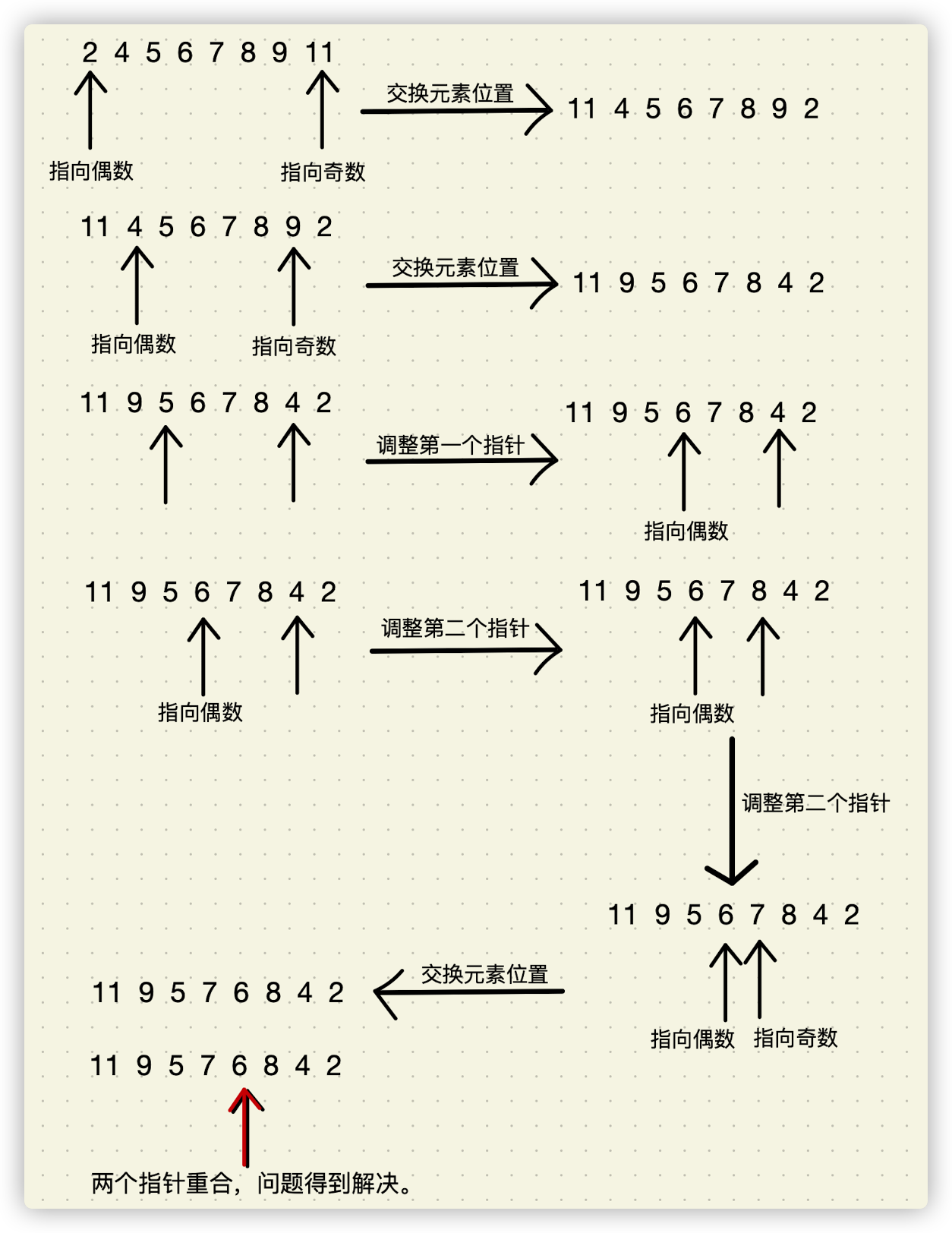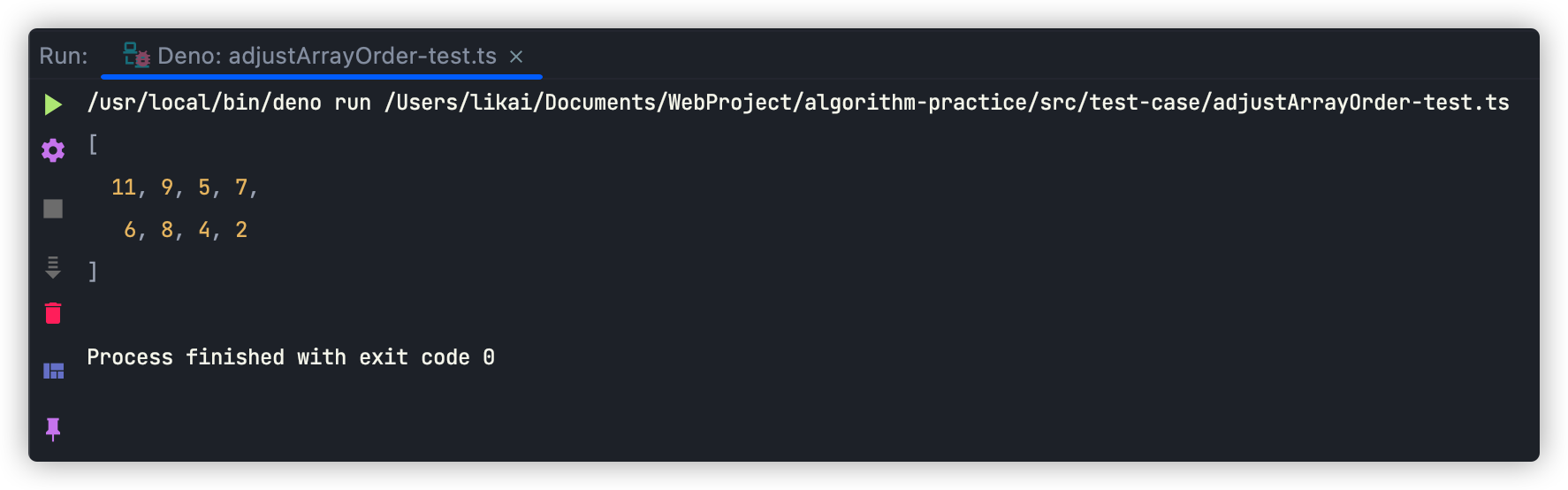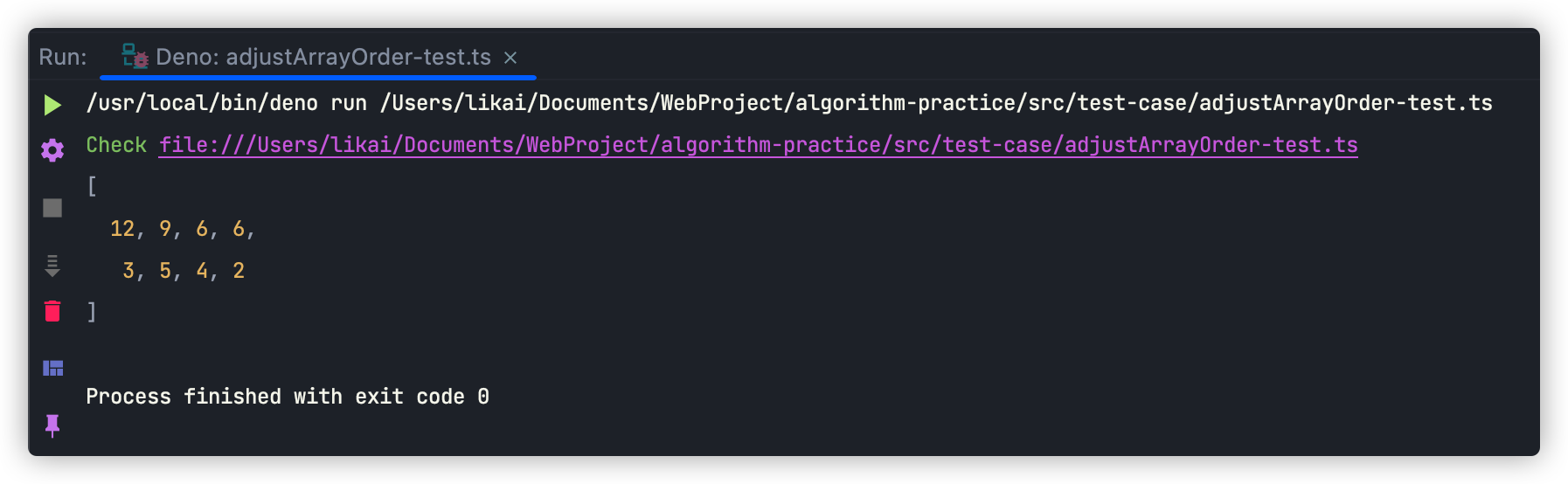• 累计撰写 165 篇文章
• 累计创建 25 个标签
• 累计收到 211 条评论

### 目 录CONTENT# 调整数组元素顺序算法2022-04-18 / 0 评论 / 10 点赞 / 557 阅读 / 2,627 字

## 实现思路

• 第一个指针初始化时指向数组的第一个数字，它只向后移动；
• 第二个指针初始化时指向数组的最后一个数字，它只向前移动；

• 第一个指针永远指向偶数，如果不为偶数就向后移动；
• 第二个指针永远指向奇数，如果不为奇数就向前移动；
• 当两个指针各自指向的数都符合条件时，就交换两个元素的位置；
• 交换完成后，重复上述步骤，直至两个指针相遇或者第一个指针位于第二个指针之后则代表问题已得到解决。## 实现代码

export class AdjustArrayOrder {
// 指向数组元素的两个指针：一个指向数组头部、一个指向数组尾部
private begin = 0;
private end = 0;

// 调整数组中奇数与偶数元素的位置：奇数位于偶数前面
reorderOddEven(arr: Array<number>): void {
this.end = arr.length - 1;
while (this.begin < this.end) {
// 向后移动begin(转成二进制跟1做与运算，运算结果为0就表示为偶数)，直至其指向偶数
while (this.begin < this.end && (arr[this.begin] & 0x1) !== 0) {
this.begin++;
}

// 向前移动end（转成二进制跟1做与运算，运算结果为1就表示为奇数），直至其指向奇数
while (this.begin < this.end && (arr[this.end] & 0x1) === 0) {
this.end--;
}

// begin指向了偶数，end指向了奇数
if (this.begin < this.end) {
// 交换两个元素的顺序
[arr[this.begin], arr[this.end]] = [arr[this.end], arr[this.begin]];
}
}
// 重置指针位置
this.begin = 0;
this.end = 0;
}
}


### 代码的可扩展性

  // 元素排序
reorder(arr: Array<number>, checkFun: (checkVal: number) => boolean): void {
this.end = arr.length - 1;
while (this.begin < this.end) {
// 向后移动begin
while (this.begin < this.end && !checkFun(arr[this.begin])) {
this.begin++;
}

// 向前移动end
while (this.begin < this.end && checkFun(arr[this.end])) {
this.end--;
}

// begin与end都指向了正确的位置
if (this.begin < this.end) {
// 交换两个元素的顺序
[arr[this.begin], arr[this.end]] = [arr[this.end], arr[this.begin]];
}
}


## 测试用例

const adjustArrayOrder = new AdjustArrayOrder();
// 奇数在前
const arr = [2, 4, 5, 6, 7, 8, 9, 11];
console.log(arr);• 负数在数组的最前面
// 负数在前
const checkMinusNumber = function (val: number) {
return val > 0;
};
const arr = [2, 4, 5, 6, 7, -8, -10 - 12, -2];
console.log(arr);• 能被3整除的数在数组的最前面
const checkDivisible = function (val: number) {
return val % 3 !== 0;
};
const arr = [2, 4, 5, 6, 3, 6, 9, 12];
console.log(arr);## 写在最后

• 文中如有错误，欢迎在评论区指正，如果这篇文章帮到了你，欢迎点赞和关注😊
• 本文首发于神奇的程序员公众号，未经许可禁止转载💌
10
• 10